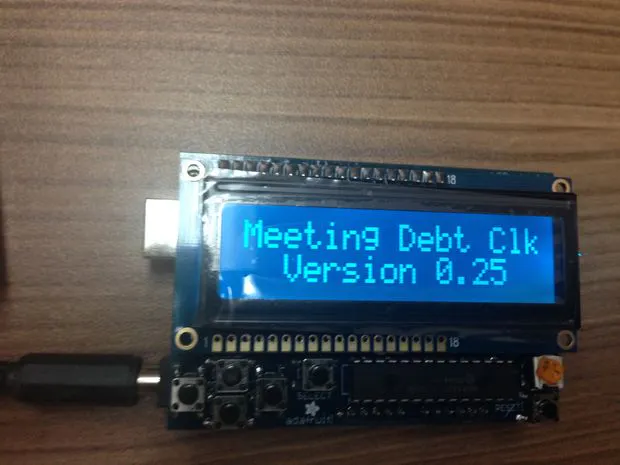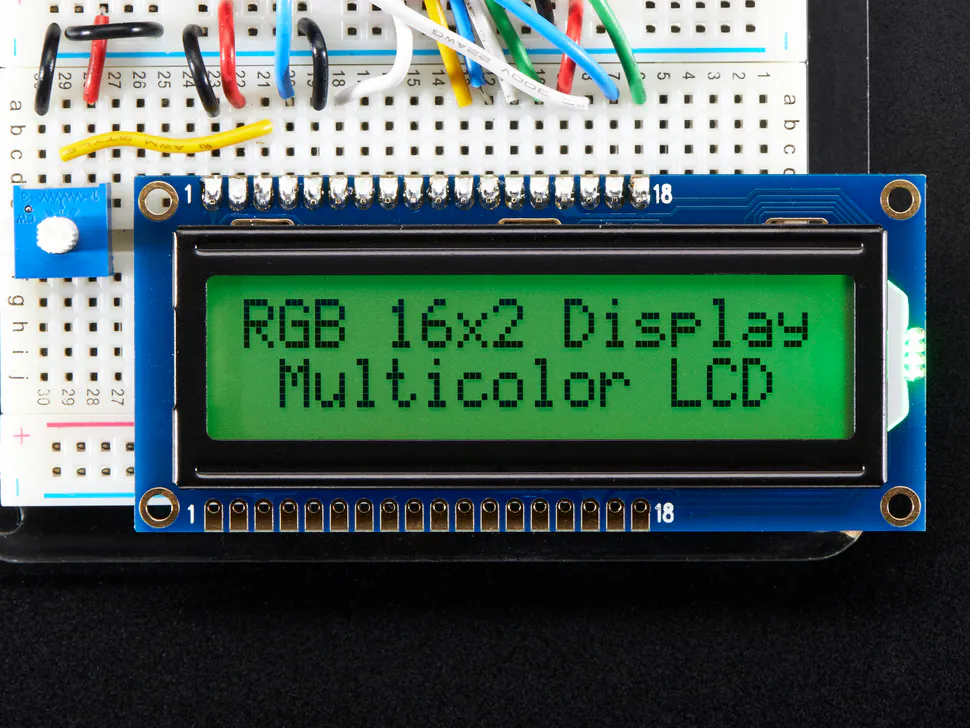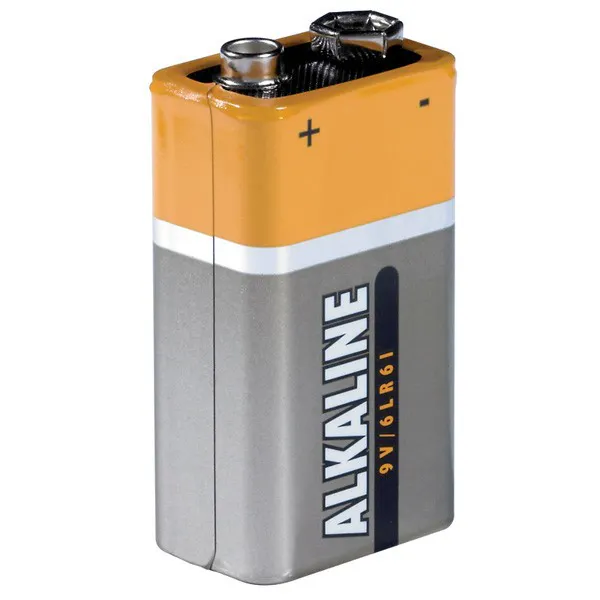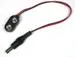# Meeting Cost Counter

See what meetings really cost.

BeginnerShowcase (no instructions)2,495## Things used in this project

### Hardware componentsAdafruit RGB Backlight LCD - 16x2
×1Arduino UNO
×19V battery (generic)
×19V to Barrel Jack Connector
×1

## Code

### Meeting Debt Clock

Arduino
```/*********************
Meeting Debt Clock
**********************/

#include <Wire.h>

#define RED 0x1
#define YELLOW 0x3
#define GREEN 0x2
#define TEAL 0x6
#define BLUE 0x4
#define VIOLET 0x5
#define WHITE 0x7
int color = 7 ;

long day = 86400000;
long hour = 3600000;
long minute = 60000;
long second =  1000;
int days = 86400000;
int hours = 3600000;
int minutes = 60000;
int seconds =  1000;

long time = 0;
long start = 0;
float salary = 0.00065 ;
int att = 0 ;
float cost = 0;
int tmr = 0 ;

void setup() {
Serial.begin(9600);
lcd.begin(16, 2);
lcd.print("Meeting Debt Clk");
lcd.setCursor(0, 1);
lcd.print("  Version 0.25 ");
lcd.setBacklight(TEAL);
}

uint8_t i=0;

void loop() {

if (att){
if (tmr > 25) {
tmr = 0 ;
cost = cost +  (salary * att) ;
display();
} else {
tmr = tmr + 1 ;
}
}

if (buttons) {
if (buttons & BUTTON_SELECT) {
color = color + 1 ;
lcd.setBacklight(color);
cost = cost +  (salary * att * 10) ;
delay (400);
}
if (buttons & BUTTON_LEFT) {
if (att > 0 ){
att = att - 1 ;
cost = cost +  (salary * att * 10) ;
display();
delay (400);
}
}
if (buttons & BUTTON_RIGHT) {
if (att == 0) {
start = millis();
cost = 0 ;
lcd.clear();
}
att = att + 1 ;
cost = cost +  (salary * att * 10) ;
display();
delay (400);
}
}
}

void display() {
time = (millis() - start) ;
days = time / day ;
hours = (time % day) / hour;
minutes = ((time % day) % hour) / minute ;
seconds = (((time % day) % hour) % minute) / second;

if (days) {
lcd.setCursor(0, 0);
lcd.print("You`ve Got To Be");
lcd.setCursor(0, 1);
lcd.print("  Kidding Me !  ");
} else {
lcd.setCursor(0, 0);
lcd.print("\$");
lcd.print (cost);
lcd.print (" ");
if (hours < 10) {
lcd.print ("0");
}
lcd.print (hours);
lcd.print (":");
if (minutes < 10) {
lcd.print ("0");
}
lcd.print (minutes);
if (cost < 1000){
lcd.print (":");
if (seconds < 10) {
lcd.print ("0");
}
lcd.print (seconds);
} else {
lcd.print("   ");
}
lcd.setCursor(0, 1);
lcd.print(att);
if (att == 1 ){
lcd.print(" Attendee ");
} else {
lcd.print(" Attendees ");
}
}
}
```

## Credits

### Robert Korn

15 projects • 21 followers
I Made the Internet of Things before people had a name for it.